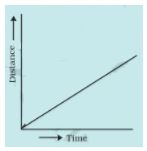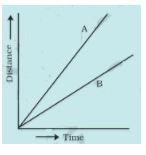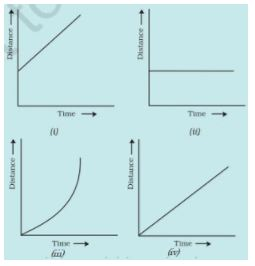# NCERT Solutions Class 7 Science Chapter 13 Motion and Time

## Chapter 13 Motion and Time Class 7 Science NCERT Solutions

Class 7 Science students should refer to the following NCERT questions with answers for Chapter 13 Motion and Time in Class 7. These NCERT Solutions with answers for Class 7 Science will come in exams and help you to score good marks

### Chapter 13 Motion and Time NCERT Solutions Class 7 Science

NCERT Solutions for Class 7 Science for chaper 13 Motion and Time

Exercises

1. Classify the following as motion along a straight line, circular or oscillatory motion:
(i) Motion of your hands while running.
(ii) Motion of a horse pulling a cart on a straight road.
(iii) Motion of a child in a merry-go-round.
(iv) Motion of a child on a see-saw.
(v) Motion of the hammer of an electric bell.
(vi) Motion of a train on a straight bridge.

(i) Oscillatory motion

(ii)Straight line motion

(iii)Circular motion

(iv) Oscillatory motion

(v)Oscillatory motion

(vi) Straight line motion

2. Which of the following are not correct?
(i) The basic unit of time is second.
(ii) Every object moves with a constant speed.
(iii) Distances between two cities are measured in kilometres.
(iv) The time period of a given pendulum is not constant.
(v) The speed of a train is expressed in m/h.

(i) Correct

(ii) Not correct
Every object may or may not moves with a constant speed.

(iii) Correct

(iv) Not correct
Time period of a given pendulum is always constant because it depends on the length of the pendulum..

(v) Not correct
The speed of a train is measured in km/h or in m/s.

3. A simple pendulum takes 32 s to complete 20 oscillations. What is the time period of the pendulum?

Numbers of oscillations = 20
Time taken = 32 sec
Time period of the pendulum = Time taken/Numbers of oscillations = 32/20 = 1.6 s.

4. The distance between two stations is 240 km. A train takes 4 hours to cover this distance. Calculate the speed of the train.

Distance between two stations = 240 km
Time taken = 4 hrs
Speed of train = Distance/Time taken = 240/4 = 60 km/hr

5. The odometer of a car reads 57321.0 km when the clock shows the time 08:30 AM. What is the distance moved by the car, if at 08:50 AM, the odometer reading has changed to 57336.0 km? Calculate the speed of the car in km/min during this time. Express the speed in km/h also.

Initial reading of odometer = 57321.0 km
Final reading of odometer = 57336.0 km
Initial Time = 8:30 AM
Final Time = 8:50 AM
Total distance covered = Final reading of odometer - Initial reading of odometer
=57336.0 - 57321.0 = 15.0 km
Total time taken = Final Time - Initial Time =  8:50 AM - 8:30 AM = 20 minutes
20 minutes = 20/60 hrs = 1/3 hrs
Speed of the car = Total distance covered/Total time taken = 15.0/20 = 0.75 km/min
Speed of the car in km/hr =15/(1/3) = 45 km/hr

6. Salma takes 15 minutes from her house to reach her school on a bicycle. If the bicycle has a speed of 2 m/s, calculate the distance between her house and the school.

Speed of bicycle = 2 m/s
Time taken = 15 mins = 15 × 60 secs = 900 secs
A/q,
Speed = Distance/Time
⇒ Distance = Speed × Time = 2 × 900 metres = 1800 metres = 1.8 km

7. Show the shape of the distance-time graph for the motion in the following cases:
(i) A car moving with a constant speed.
(ii) A car parked on a side road.

(i)The graph will be a straight line passing through the origin.(ii) The graph is a straight line parallel to x-axis or time axis.8. Which of the following relations is correct?
(i) Speed = Distance × Time           (ii) Speed = Distance/Time
(iii) Speed = Time/Distance           (iv) Speed = 1/Distance × Time

(ii) Speed = Distance/Time

9. The basic unit of speed is:
(i) km/min                    (ii) m/min
(iii) km/h                      (iv) m/s

(iv) m/s

10. A car moves with a speed of 40 km/h for 15 minutes and then with a speed of 60 km/h for the next 15 minutes. The total distance covered by the car is:
(i) 100 km                   (ii) 25 km
(iii) 15 km                   (iv) 10 km

(ii) 25 km

Firstly, the car is moving with 40km/h for 15 minutes.

15 minutes = 15/60 hrs = 1/4 hrs
Distance covered = 40 × 1/4 = 10 km
Secondly, the car is moving with 60km/h for 15 minutes.Distance covered = 60 × 1/4 = 15 km

Total distance covered = 10 km + 15 km = 25 km

11. Suppose the two photographs, shown in Fig. 13.1 and Fig. 13.2, had been taken at an interval of 10 seconds. If a distance of 100 metres is shown by 1 cm in these photographs, calculate the speed of the blue car.First, measure the distance with the help of scale and then proceed as given below.
Suppose the distance measured by scale is 2cm.
Multiply 2 with 100 to get the actual distance = 2 × 100 = 200 m.    (1cm = 100m)
Interval time between the photos taken = 10 s.
Speed of the blue car = 200/10 m/s = 20 m/s

12. Fig. 13.15 shows the distance-time graph for the motion of two vehicles A and B. Which one of them is moving faster?Vehicle A is moving faster because it has more slope than vehicle B. In distance-time graph, speed is measured by its slope.

13. Which of the following distance-time graphs shows a truck moving with speed which is not constant?Option (iii) because the slope of the graph is not a straight line and hence it does not show a uniform motion.

 NCERT Solutions Class 7 Science Chapter 1 Nutrition in Plants
 NCERT Solutions Class 7 Science Chapter 2 Nutrition in Animals
 NCERT Solutions Class 7 Science Chapter 3 Fibre to Fabric
 NCERT Solutions Class 7 Science Chapter 4 Heat
 NCERT Solutions Class 7 Science Chapter 5 Acids Bases and Salts
 NCERT Solutions Class 7 Science Chapter 6 Physical and Chemical Changes
 NCERT Solutions Class 7 Science Chapter 7 Weather Climate and Adaptations of Animals to Climate
 NCERT Solutions Class 7 Science Chapter 8 Winds Storms and Cyclones
 NCERT Solutions Class 7 Science Chapter 9 Soil
 NCERT Solutions Class 7 Science Chapter 10 Respiration in Organisms
 NCERT Solutions Class 7 Science Chapter 11 Transportation in Animals and Plants
 NCERT Solutions Class 7 Science Chapter 12 Reproduction in Plants
 NCERT Solutions Class 7 Science Chapter 13 Motion and Time
 NCERT Solutions Class 7 Science Chapter 14 Electric Current and its Effects
 NCERT Solutions Class 7 Science Chapter 15 Light
 NCERT Solutions Class 7 Science Chapter 16 Water A Precious Resource
 NCERT Solutions Class 7 Science Chapter 17 Forests Our Lifeline
 NCERT Solutions Class 7 Science Chapter 18 Wastewater Story

## More Study Material

Tags

### NCERT Solutions Class 7 Science Chapter 13 Motion and Time

NCERT Solutions Class 7 Science Chapter 13 Motion and Time is available on our website www.studiestoday.com for free download in Pdf. You can read the solutions to all questions given in your Class 7 Science textbook online or you can easily download them in pdf.

### Chapter 13 Motion and Time Class 7 Science NCERT Solutions

The Class 7 Science NCERT Solutions Chapter 13 Motion and Time are designed in a way that will help to improve the overall understanding of students. The answers to each question in Chapter 13 Motion and Time of Science Class 7 has been designed based on the latest syllabus released for the current year. We have also provided detailed explanations for all difficult topics in Chapter 13 Motion and Time Class 7 chapter of Science so that it can be easier for students to understand all answers.

#### NCERT Solutions Chapter 13 Motion and Time Class 7 Science

Class 7 Science NCERT Solutions Chapter 13 Motion and Time is a really good source using which the students can get more marks in exams. The same questions will be coming in your Class 7 Science exam. Learn the Chapter 13 Motion and Time questions and answers daily to get a higher score. Chapter 13 Motion and Time of your Science textbook has a lot of questions at the end of chapter to test the students understanding of the concepts taught in the chapter. Students have to solve the questions and refer to the step-by-step solutions provided by Science teachers on studiestoday to get better problem-solving skills.

#### Chapter 13 Motion and Time Class 7 NCERT Solution Science

These solutions of Chapter 13 Motion and Time NCERT Questions given in your textbook for Class 7 Science have been designed to help students understand the difficult topics of Science in an easy manner. These will also help to build a strong foundation in the Science. There is a combination of theoretical and practical questions relating to all chapters in Science to check the overall learning of the students of Class 7.

#### Class 7 NCERT Solution Science Chapter 13 Motion and Time

NCERT Solutions Class 7 Science Chapter 13 Motion and Time detailed answers are given with the objective of helping students compare their answers with the example. NCERT solutions for Class 7 Science provide a strong foundation for every chapter. They ensure a smooth and easy knowledge of Revision notes for Class 7 Science. As suggested by the HRD ministry, they will perform a major role in JEE. Students can easily download these solutions and use them to prepare for upcoming exams and also go through the Question Papers for Class 7 Science to clarify all doubts

Where can I download latest NCERT Solutions for Class 7 Science Chapter 13 Motion and Time

You can download the NCERT Solutions for Class 7 Science Chapter 13 Motion and Time for latest session from StudiesToday.com

Can I download the NCERT Solutions of Class 7 Science Chapter 13 Motion and Time in Pdf

Yes, you can click on the link above and download NCERT Solutions in PDFs for Class 7 for Science Chapter 13 Motion and Time

Are the Class 7 Science Chapter 13 Motion and Time NCERT Solutions available for the latest session

Yes, the NCERT Solutions issued for Class 7 Science Chapter 13 Motion and Time have been made available here for latest academic session

How can I download the Chapter 13 Motion and Time Class 7 Science NCERT Solutions

You can easily access the links above and download the Chapter 13 Motion and Time Class 7 NCERT Solutions Science for each chapter

Is there any charge for the NCERT Solutions for Class 7 Science Chapter 13 Motion and Time

There is no charge for the NCERT Solutions for Class 7 Science Chapter 13 Motion and Time you can download everything free

How can I improve my scores by reading NCERT Solutions in Class 7 Science Chapter 13 Motion and Time

Regular revision of NCERT Solutions given on studiestoday for Class 7 subject Science Chapter 13 Motion and Time can help you to score better marks in exams

Are there any websites that offer free NCERT solutions for Chapter 13 Motion and Time Class 7 Science

Yes, studiestoday.com provides all latest NCERT Chapter 13 Motion and Time Class 7 Science solutions based on the latest books for the current academic session

Can NCERT solutions for Class 7 Science Chapter 13 Motion and Time be accessed on mobile devices

Yes, studiestoday provides NCERT solutions for Chapter 13 Motion and Time Class 7 Science in mobile-friendly format and can be accessed on smartphones and tablets.

Are NCERT solutions for Class 7 Chapter 13 Motion and Time Science available in multiple languages

Yes, NCERT solutions for Class 7 Chapter 13 Motion and Time Science are available in multiple languages, including English, Hindi